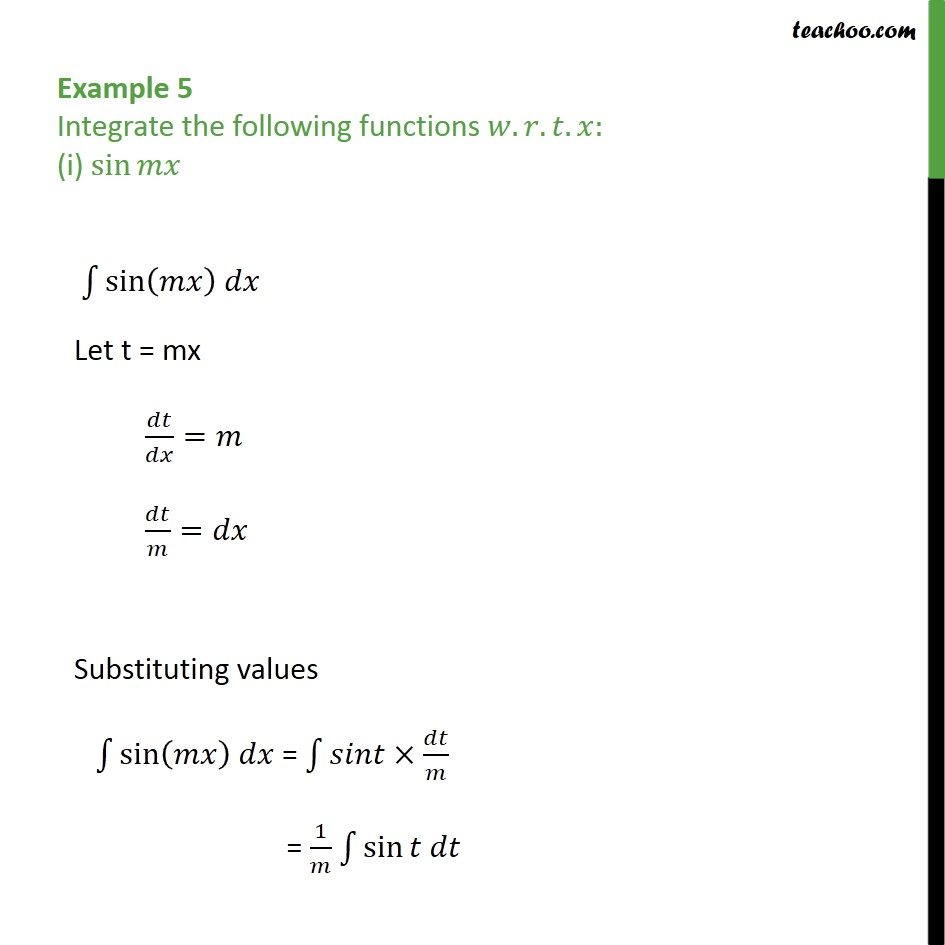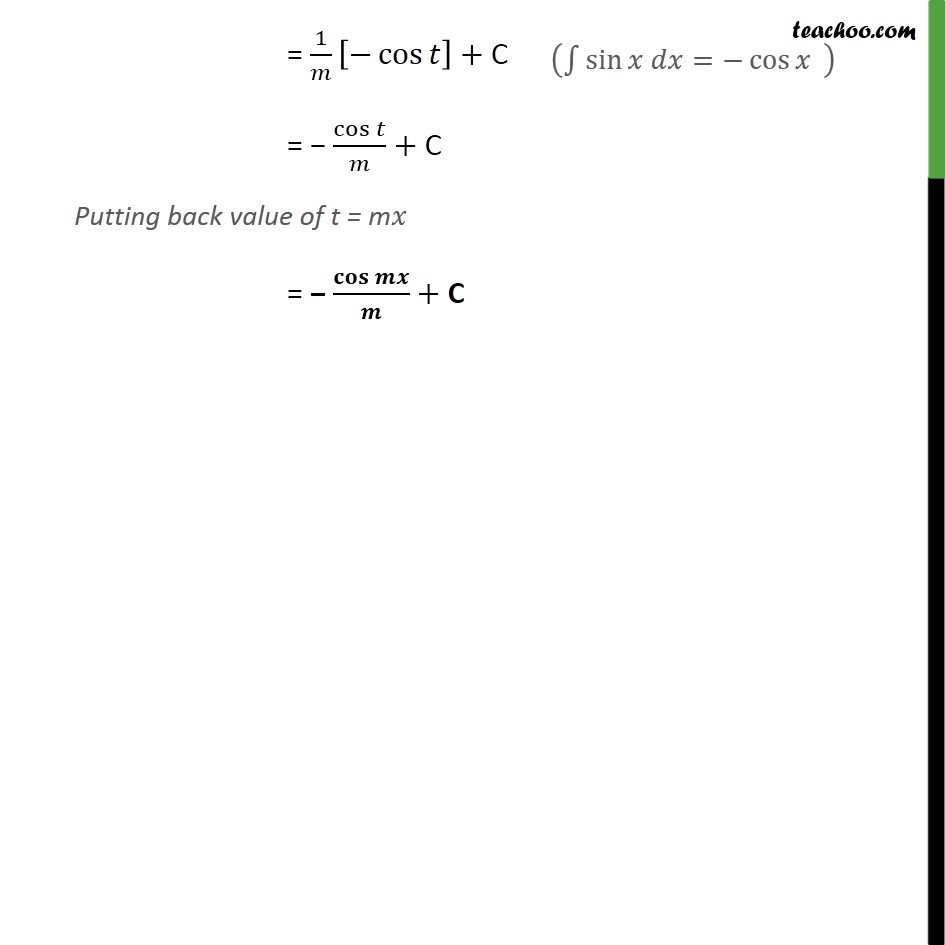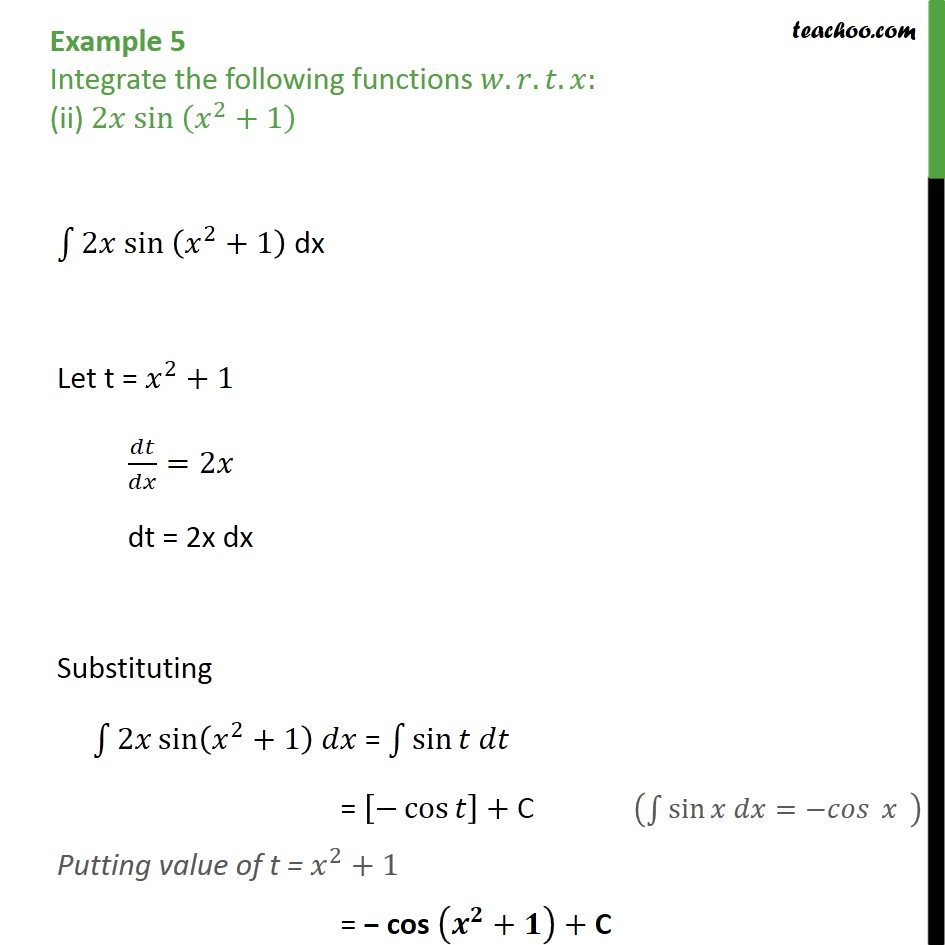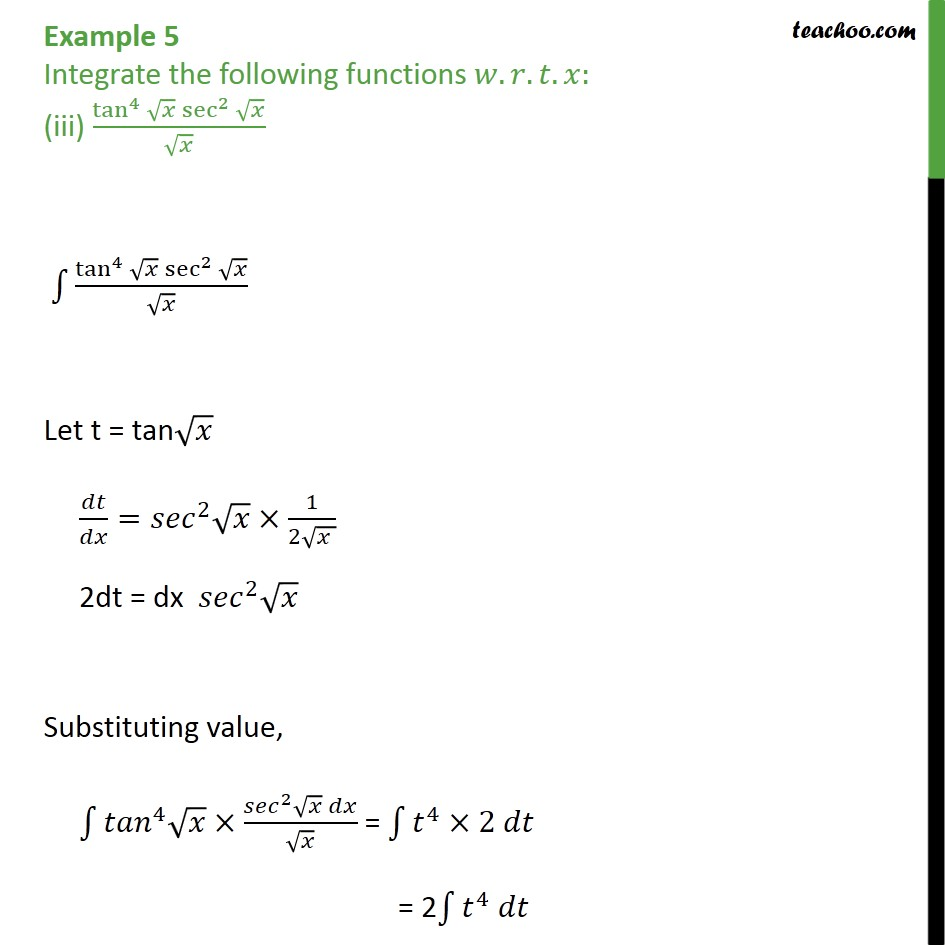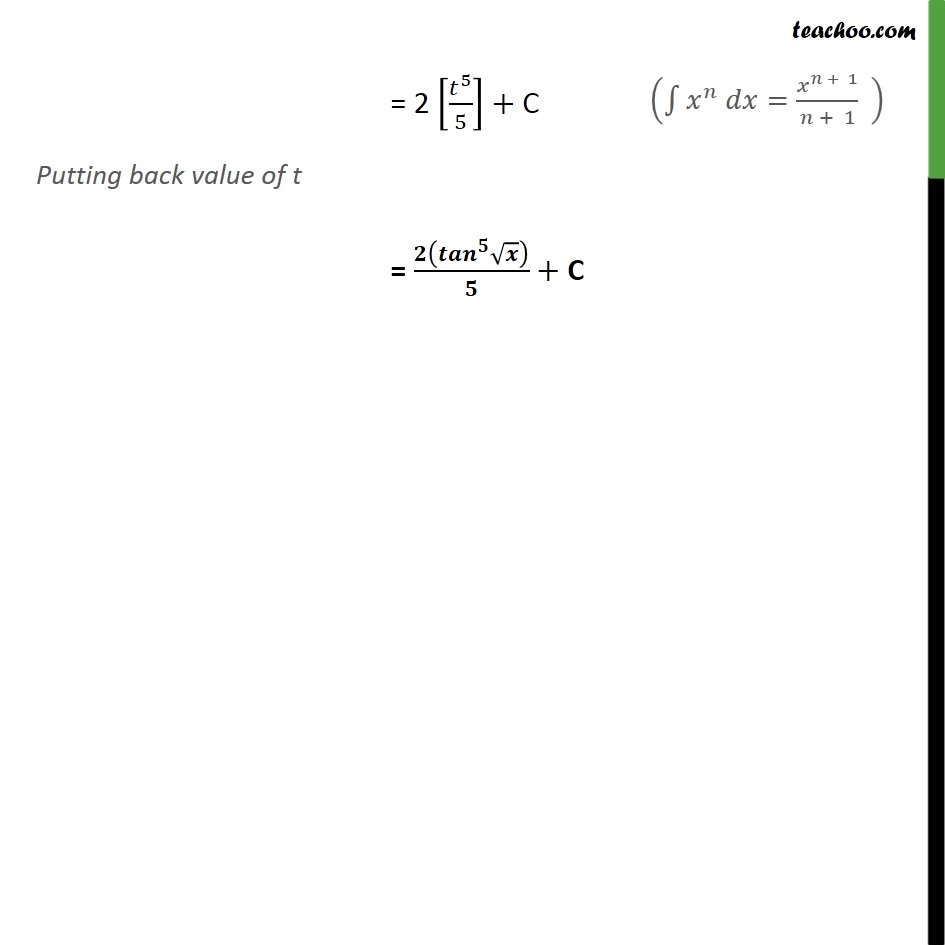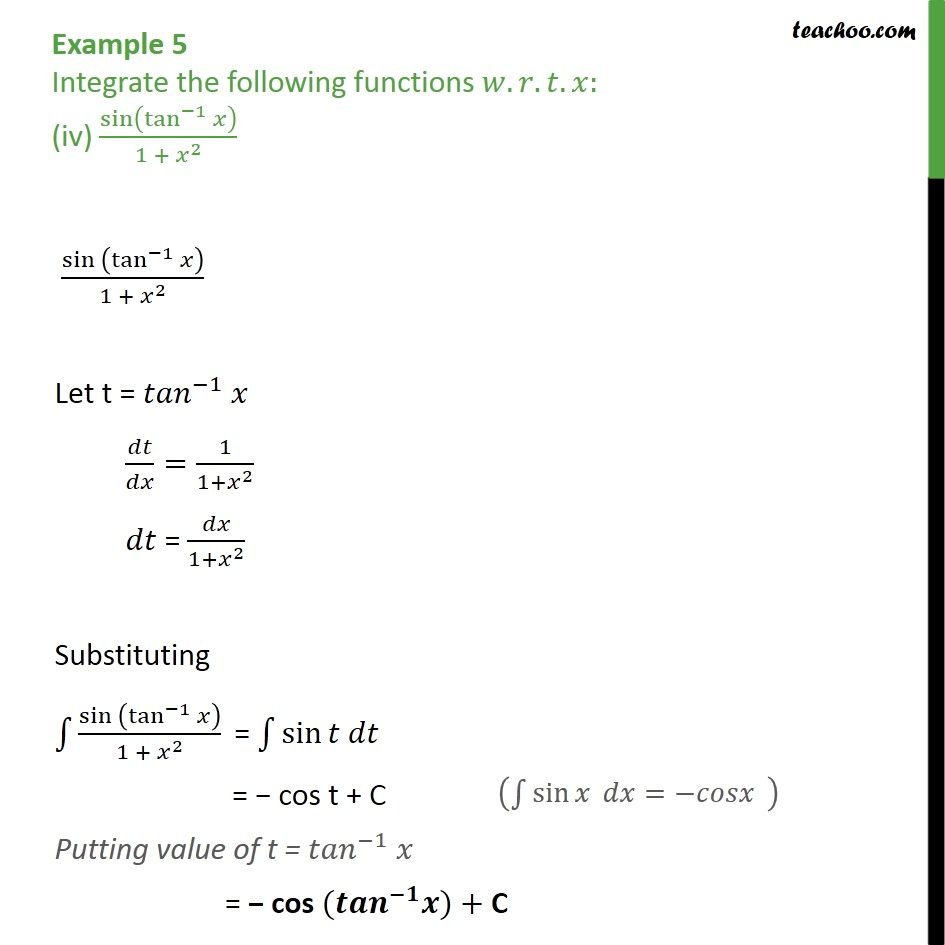1. Chapter 7 Class 12 Integrals
2. Concept wise
3. Integration by substitution - Trignometric - Normal

Transcript

Example 5 Integrate the following functions . . . : (i) sin sin Let t = mx = = Substituting values sin = = 1 sin = 1 sin = 1 cos + C = cos + C Putting back value of t = m = + C Example 5 Integrate the following functions . . . : (ii) 2 sin 2 +1 2 sin 2 +1 dx Let t = 2 +1 =2 dt = 2x dx Substituting 2 sin 2 +1 = sin = cos + C Putting value of t = 2 +1 = cos + + C Example 5 Integrate the following functions . . . : (iii) tan 4 sec 2 tan 4 sec 2 Let t = tan = 2 1 2 2dt = dx 2 Substituting value, 4 2 = 4 2 = 2 4 = 2 5 5 + C Putting back value of t = 2 5 5 + C Example 5 Integrate the following functions . . . : (iv) sin tan 1 1 + 2 sin tan 1 1 + 2 Let t = 1 = 1 1+ 2 = 1+ 2 Substituting sin tan 1 1 + 2 = sin = cos t + C Putting value of t = 1 = cos ( 1 )+ C

Integration by substitution - Trignometric - Normal